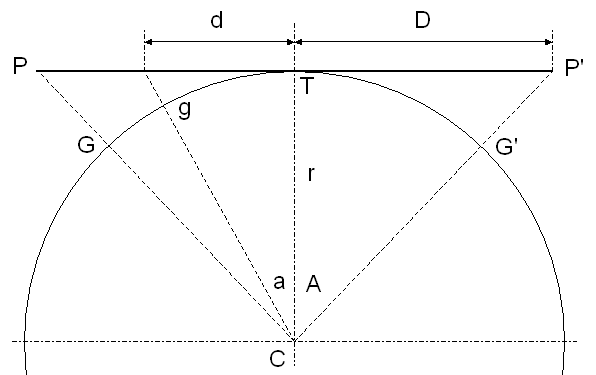# Panoramas & overlapping photos

In the following diagram (a plan view) two objects, A and B, are photographed twice by camera C when it is pointing in different directions. The camera records the objects on the geometric plane of its detector (or film) and this image will remain as a flat plane when it is displayed or printed.It is fairly obvious that the distance between the images of A and B is different along the blue line, which is the plane of photo 1 seen edge-on, from the distance in the red plane of photo 2. So if we want to combine the two overlapping images to make one panoramic view, some stretching or squeezing is going to be necessary. Furthermore, though less obviously, the amount of stretching/squeezing is a variable factor, changing with distance from the centre of each photograph.

However, if we had imaged on a sphere (the cross-section of which is shown as a circle in the diagram above), the direction of pointing the camera would be irrelevant. The distance between the images of any two objects would then be constant regardless of the camera direction.

So if we project each photograph onto a sphere it will be possible to superimpose them without any other distortion. The type of projection we need is called "inverse gnomonic". A gnomonic projection projects from a spherical surface to a flat plane, so we want the inverse of that.

### Gnomonic projection

A good mathematical description of the gnomonic projection can be found at Wolfram MathWorld. The following gives details of the maths used in our implementation of the projection in Java class Gnomonic.In this diagram photo PP' is width 2 x D, of a scene subtending angle 2 x A at camera C. The photo is tangential at T to sphere radius r centred on C. Inverse gnomonic projection onto the sphere plots the photo as GG'. General point distance d from T maps to g in this projection, making angle a at C.

We can measure A, as half the angular field of view of the particular lens used for taking the photo.

tan A = D / r => r = D / tan A
arc TG = A . r => arc TG = A . D / tan A
tan a = d / r => a = arctan (d / r)
arc Tg = a . r => arc Tg (d) = arctan (tan A . d / D) . D / tan ATo transform point (x, y) in the photo:

d = sqrt (x . x + y . y)
d' = arctan (tan A . d / D) . D / tan A
x' = d' . x / d
y' = d' . y / d

to make the inverse gnomonic projection, mapping the plane photo onto the surface of the sphere.

In practice the inverse projection will be done by working backwards because of interpolating between source pixels. So we need the forward projection after all, from point (x', y') on the sphere:

d' = sqrt (x' . x' + y' . y')
d = D . tan (tan A . d' / D) / tan A
x = x' . d / d'
y = y' . d / d'

We then know (x, y), the point to sample from (which has non-integer coordinates, so interpolate) in order to make point (x',y') in the target image.

Notice that the calculations need the angle A but not the radius r. We do not need to know how far away are the objects being photographed.

The projection is important when the angle is large, such as 30 degrees, but will not be necessary for photographs taken through a telescope where the angle is a degree or less. We do not yet know where the boundary lies: what is the smallest angle A for which it is necessary to do the projection for successfully matching of overlapping photos?

### Angle of view for a camera lens

Before using our implementation it is necessary to know the half-angle of the field of view, both horizontally (angle Ah) and vertically (Av). These angles can be calculated from the focal length of the lens if we know the width (w) and height (h) of the camera detector or film frame:

Ah = arctan (w / 2f)

Av = arctan (h / 2f)

For a 35mm film camera w = 36mm and h = 24mm. Some of the more expensive digital SLR's have the same dimensions but others have smaller detectors. Check the documentation for your particular camera and enter the width and height via GRIP's configuration menu.

GRIP can usually obtain the focal length in mm which was used for a particular photograph by reading metadata in the image file. If the focal length is not present in the metadata it will ask the user for the value.

The author does not know how accurately lens manufacturers quote the focal length, nor how accurate it needs to be for our purposes (particularly the gnomonic projection for merging overlapping photos). It would be a good idea to make several experimental measurements with different focal lengths and lenses, photographing a scale from a known distance.

Next page